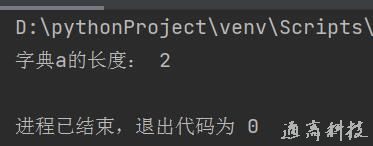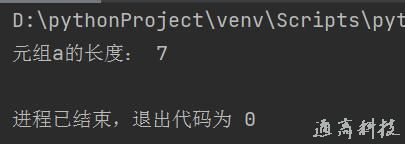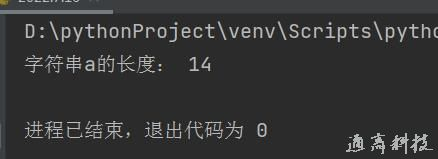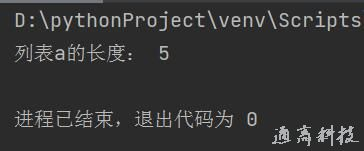# python中len()函数的用法

python中len()主要用于对字符、列表、元组的长度计算，传入值返回对象的长度

### python中len()语法

len( s )

s:需要计算的字符串、列表、字典、元组等

### python中len()计算字典长度

1. a= {'name':'通高科技','age':18}

2. print('字典a的长度：',len(a))### python中len()计算元组长度

1. a=(1,2,3,4,5,6,7)

2. print('元组a的长度：',len(a))### python中len()计算字符串长度

1. a='tonggao001.com'

2. print('字符串a的长度：',len(a))### python中len()计算列表长度

1. a=[1,2,3,4,5]

2. print('列表a的长度：',len(a))